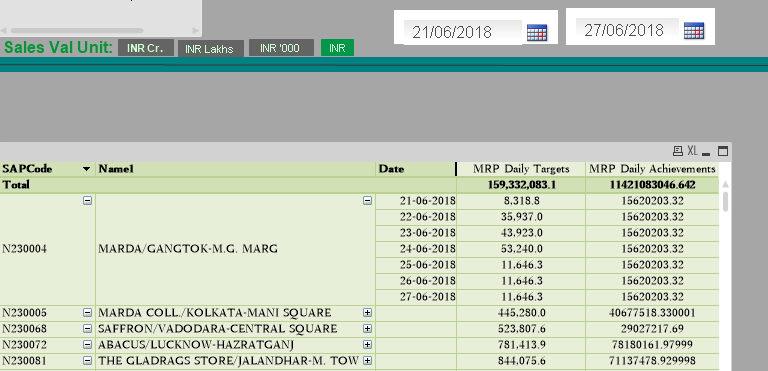# QlikView App Dev

Discussion Board for collaboration related to QlikView App Development.

Announcements
Action-Packed Learning Awaits! QlikWorld 2023. April 17 - 20 in Las Vegas: REGISTER NOW
cancel
Showing results for
Did you mean:Creator II

## set analysis for starting to end date

HI,

I want when user selects the date in calendar then  budget(MRP daily target) and sales (Mrp daily achievement) should be displayed.

here i am getting same values for daily sales for period 21/6/2018 to 27/06/2018 which is not correct, please help stalwar1

following is the expression for MRP daily target

=num(sum({ <BugetDate = {">=\$(From)<=\$(To)"} >}distinct Budget),'#,##0.0')

following is the expression for MRP daily achievements

=num(sum({<BugetDate = {">=\$(From)<=\$(To)"} >}[inv value]/Sales_INR_Unit))3 RepliesSpecialist III

Hi, try with :

=num(sum({ <BugetDate = {">=\$(=From) <=\$(=To)"} >}distinct Budget),'#,##0.0')

=num(sum({<BugetDate = {">=\$(=From) <=\$(=To)"} >}[inv value]/Sales_INR_Unit))Champion III

CCan you share your app to look into if possible and your expected output values for those expressions?Creator II
Author

This problem was solved by using following expression

=num(sum({< Sold_Date = {">=\$(From)<=\$(To)"} >} Budget),'#,##0.0')Community Browser